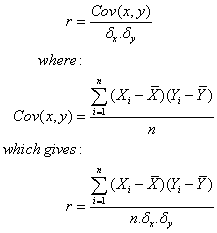## Thursday, January 17, 2008

### Calculating correlation co-efficients

The correlation co-efficient for a set of pairs of data provides a measure of the strength and direction (positive or negative) of the linear relationship between two variables. The most commonly used is the Pearson correlation co-efficient, which uses a least squares method of calculating the dispersion of the data pairs from a theoretical straight-line (linear) relationship.

The correlation co-efficient, r, for a set of (x,y) data pairs is calculated as follows:The following steps can be followed:
1. Calculate the average values for both x (x*) & y (y*);
2. For each row, calculate (x – x*) and (y – y*);
3. For each row, calculate (x-x*)2, (y – y*)2, and (x-x*)(y-y*);
4. Add up the values in each column, and store the totals
5. For both x & y values, calculate the standard deviation, sx and sy, using the totals for (x-x*)2 and (y-y*)2 dividing each by the number of rows, and taking the square root of the results.
6. Calculate r using the total for the column of (x-x*)(y-y*) and dividing by (n*sx*sy) where n is the number of rows in the table (i.e. the number of x,y pairs.

The following table should help to illustrate the calculation:
 x y (x-x*) (x-x*)2 (y-y*) (y-y*)2 (x-x*)(y-y*) 1 8.56 -4 16 1.865556 3.480297531 -7.46222222 2 8.23 -3 9 1.535556 2.357930864 -4.60666667 3 7.62 -2 4 0.925556 0.856653086 -1.85111111 4 7.12 -1 1 0.425556 0.181097531 -0.42555556 5 6.99 0 0 0.295556 0.087353086 0 6 7.05 1 1 0.355556 0.126419753 0.355555556 7 4.98 2 4 -1.71444 2.939319753 -3.42888889 8 5.37 3 9 -1.32444 1.754153086 -3.97333333 9 4.33 4 16 -2.36444 5.590597531 -9.45777778 mean x* 5 Total 60 17.37382222 -30.85 mean y* 6.694444 std dev 2.581989 1.38939724 r= -0.9555

In the above table, the x values represent the number of guests; and the y values represent the conversion rate given as a percentage. The columns headed by (x-x*)2 and (y-y*)2 are used in the calculation of the standard deviations for x and y – sx and sy. Once the last column is calculated, the values are totaled, giving the numerator (upper value of the fraction) in the equation for r.

For the above example, r is calculated as:

The use of the correlation co-efficient enables a determination as to whether or not there exists a relationship between the variables. A strong correlation does not indicate a causal relationship in the data; although causal relationships show strong correlation.

Note: the value for the correlation co-efficient r can range from -1 to 1. A value towards either end of the range indicates a strong correlation between the variables; values close to 0 indicate very little or no correlation.

#### 1 comment:

BasiaBernstein said...

Correlation is computed into what is known as the correlation coefficient, which ranges between -1 and +1. Perfect positive correlation (a correlation co-efficient of +1) implies that as one security moves, either up or down, the other security will move in lockstep, in the same direction.
perason correlation